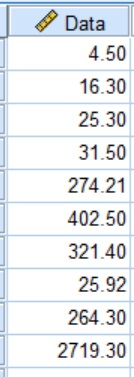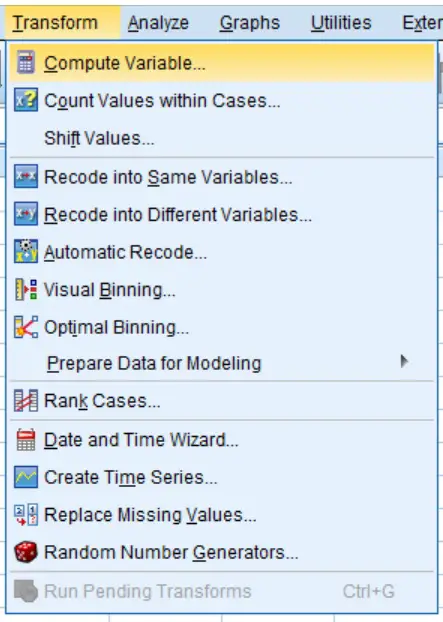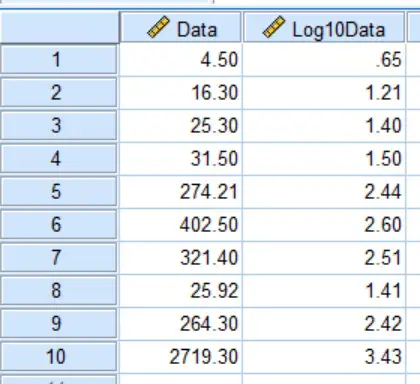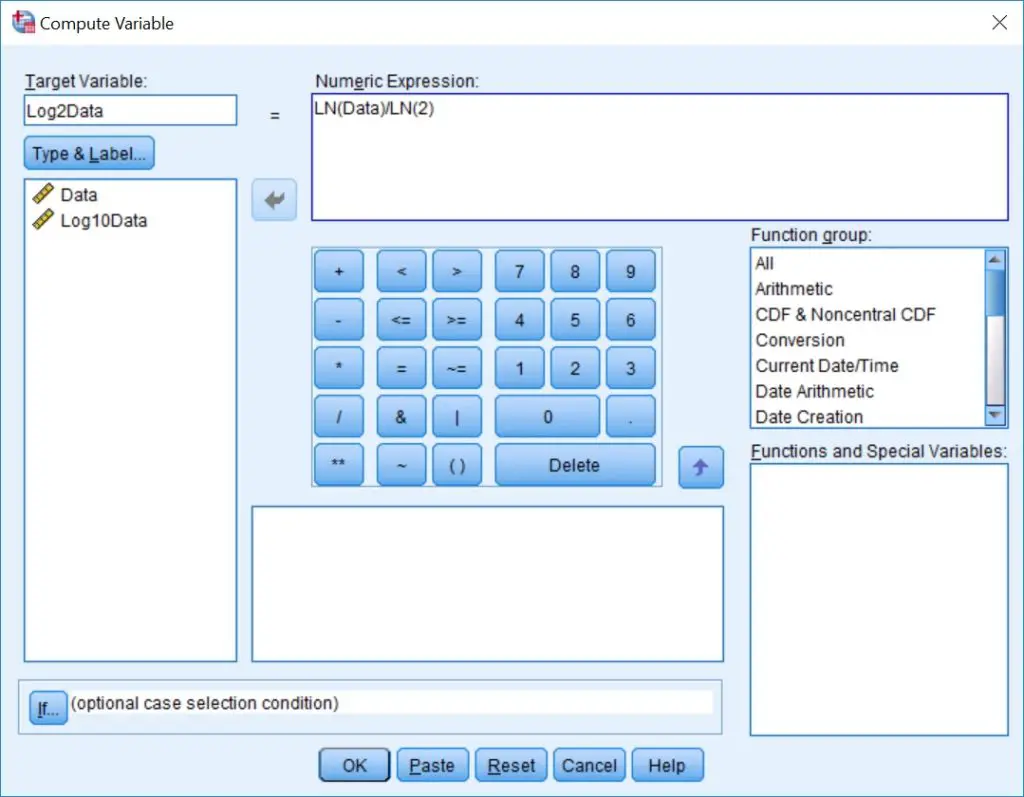How To Log Transform Data In SPSS

In this guide, I will show you how to log (log10) transform data in SPSS. I will also describe how to log transform data with a base other than 10.

What is a log transformation?

Log transformation is used when data is highly skewed. Usually, log transformation is performed with a base of 10, hence the term ‘log10‘.

Understanding log transformation is best seen with an example. Let’s say we want to log10 transform the number ‘100‘. In other words, the base number (i.e 10 in this case) to the power of what number is needed to make 100? Since, 10 x 10 (i.e. 102) equals 100, then we can say log10(100) = 2.

Taking another example to elaborate on how log10 works, let’s work out what log10(1,000) is. Since 10 x 10 x 10 (i.e. 103) equals 1,000, then we can say log10(1,000) = 3.

How to log (log10) transform data in SPSS

To log (log10) transform data in SPSS, simply follow the steps below. In this example, I have a variable containing 10 numbers called ‘Data‘.1. In SPSS, go to ‘Transform > Compute Variable …‘.2. In the ‘Compute Variable‘ window, enter the name of the new variable to be created in the ‘Target Variable‘ box, found in the upper-left corner of the window. I suggest calling this ‘Log10X‘, with X being the name of the original variable. In this example, my variable to be transformed is called ‘Data‘, so I am calling the newly transformed variable ‘Log10Data‘.

Then in the ‘Numeric Expression‘ window, type in ‘LG10(X)‘, with X being replaced with the name of the variable to be transformed. So, in this example, I will enter ‘LG10(Data)‘.Then click the ‘OK‘ button to transform the data.

The output

A new variable should now be present in your data sheet. This will be the newly log10 transformed data. Here is what my datasheet now looks like.How to log transform data with a different base in SPSS

Unfortunately, there isn’t a direct function to log transform data with a base other than 10 in SPSS. However, there is a way to do this by creating a formula. I will now show you how to do this.

1. Go to the ‘Compute Variable‘ window again by selecting ‘Transform > Compute Variable …‘.

2. Now it’s time to write the formula. Let’s say I want to log transform a variable with a base of 2 (instead of 10).

To do this, I will enter ‘LN(Data)/LN(2)‘ into the ‘Numeric Expression‘ window. Obviously, replace ‘Data‘ with the name of the variable to be transformed. Also, replace the ‘2‘ with the base of any other number that you want to log transform with.

Also, don’t forget to fill in the ‘Target Variable‘ box with the name of the new variable that will be created. In this example, I am calling the variable ‘Log2Data‘.Finally, click the ‘OK‘ button to transform the data.

Conclusion

In this article, I have explained step-by-step how to log transform data in SPSS. Usually, this is performed with the base 10, using the function ‘LG10()‘. However, other bases can be used in the log transformation by using the formula ‘LN()/LN(base)‘, where the base can be replaced with the desired number.

SPSS version used: 25